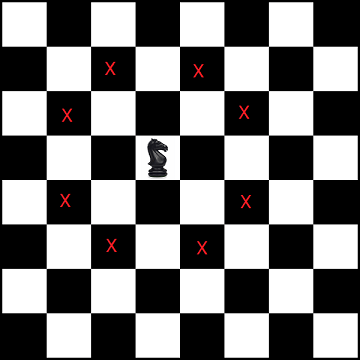# Chess Knight Problem | Find Shortest path from source to destination

Given a chess board, find the shortest distance (minimum number of steps) taken by a Knight to reach given destination from given source.

For example,

Input: N = 8 (8 x 8 board), Source = (7, 0) Destination  = (0, 7)

Output: Minimum number of steps required is 6

Explanation: The Knight’s movement can be demonstrated in figure belowThe idea is to use Breadth First Search (BFS) as it is a Shortest Path problem. Below is the complete algorithm.

1. Create an empty queue and enqueue source cell having
distance 0 from source (itself)

2. do till queue is not empty

a) Pop next unvisited node from queue

b) If the popped node is destination node, return its distance

c) else we mark current node as visited and for each of 8 possible
movements for a knight, we enqueue each valid movement into the
queue with +1 distance (min distance of given node from source
= min distance of parent from source + 1)

A knight can move in 8 possible directions from a given cell as illustrated in below figure –We can find all the possible locations the Knight can move to from the given location by using the array that stores the relative position of Knight movement from any location. For example, if the current location is (x, y), we can move to (x + row[k], y + col[k]) for 0 <= k <=7 using below array.

row[] = [ 2, 2, -2, -2, 1, 1, -1, -1 ]
col[] = [ -1, 1, 1, -1, 2, -2, 2, -2 ]

So, from position (x, y) Knight’s can move to:

(x + 2, y – 1)
(x + 2, y + 1)
(x – 2, y + 1)
(x – 2, y – 1)
(x + 1, y + 2)
(x + 1, y – 2)
(x – 1, y + 2)
(x – 1, y – 2)

Note that in BFS, all cells having shortest path as 1 are visited first, followed by their adjacent cells having shortest path as 1 + 1 = 2 and so on.. so if we reach any node in BFS, its shortest path = shortest path of parent + 1. So, the first occurrence of the destination cell gives us the result and we can stop our search there. It is not possible that the shortest path exists from some other cell for which we haven’t reached the given node yet. If any such path was possible, we would have already explored it.

## C++

Output:

Minimum number of steps required is 6

## Java

Output:

Minimum number of steps required is 6

Exercise: Extend the solution to print the paths as well.(3 votes, average: 5.00 out of 5)Loading...

Please use our online compiler to post code in comments. To contribute, get in touch with us.
Like us? Please spread the word and help us grow. Happy coding 🙂Subscribe
Notify ofGuest

Great stuff! There is also a variation of this problem where you calculate the minimum number of moves to get to a position in an infinite coordinate plane using a formula in constant time (there is some hard coding involved for values within 4 spaces of the origin or so. Any plans to attack that one?Guest
pratik Sharma

Can someone please explain this bunchGuest

Thanks, I have found your website to be very useful… especially the codes are neat and well explained.Guest

great!Guest

“Exercise: Extend the solution to print the paths as well.”
What is the answer?Guest

Interesting that you kept track of visited/unvisited marks. I had used similar approach of using the closest point approach for moving the knight in the beginning. But as the knight reaches a proximity distance (which is very close to the target), I used a different set of algorithm.

Please look into my solution and let me know what you think:

http://sovitpoudel.com.np/2016/06/03/a-knights-watch/

Thanks!Guest
Alexis Lozano Terán

if I want to solve in o(1) ?Guest
Robert Word

My friends, here is the closed form solution. Represent the desired shortest knight path by the Gaussian integer g. The general path achieving this displacement can be written:

g = ( (1-i)g + (2-i)d)(2+i) – (g+(2+i)d)(2-i) where g is the desired displacement of the chess knight expressed as a Gaussian integer, and d is selected to minimize the number of Knight moves by setting

d = Cint( g(2i-5)/10); Clint is “closest Gaussian integer.

This provides a closed form solution.